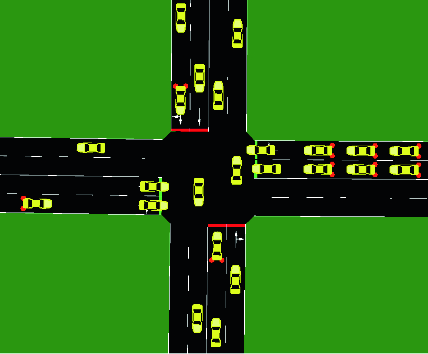#### Optimal Shielding in Urban Traffic Control

[Avni et al.] synthesize optimal shields that overwrite the commands of a traffic-light controller modeled in the traffic simulator SUMO. See Figure 1 for an example traffic junction. The optimal-shield needs to balance the number of waiting cars per incoming road with the cost for interfering with the traffic light controller. The example is parametrised with the cut-off parameter k that defines the maximal modelled number of waiting cars per road.#### Model Specification

We discuss the model given in Figure 2 in detail and describe the different sections of the code. The property used to synthesise the optimal shield is given in Figure 3.

The keyword smg defines the model to be a stochastic multiplayer game.

At first we define the actions controllable by each player with the player...endplayer struct. Square brackets indicate an action to be controlled by the described player. Simple strings add all unlabeled actions of the given module to the player struct.

The global variable move models the turn-based character of the coalition game. It is switched between the controller, the shield and the environment such that they move in a round-trip manner.

We then declare an undefined constant lmax which serves as the cut-off parameter of the variables N, E, S and W.

```smg

player controller
cont
endplayer

player shield
[reduceNS_Shield], [reduceEW_Shield], [reduceNS_ShieldDev], [reduceEW_ShieldDev]
endplayer

player environment
env
endplayer

global move : [0..2];

const int lmax;

global N : [0..lmax];
global E : [0..lmax];
global S : [0..lmax];
global W : [0..lmax];

module env
[] move=0 -> 1/8 : (N'=min(lmax,N+1)) & (move'=1) +
1/8 : (S'=min(lmax,S+1)) & (move'=1) +
1/8 : (E'=min(lmax,E+1)) & (move'=1) +
1/8 : (W'=min(lmax,W+1)) & (move'=1) +

7/120 : (N'=min(lmax,N+1)) & (S'=min(lmax,S+1)) & (move'=1) +
7/120 : (S'=min(lmax,S+1)) & (W'=min(lmax,W+1)) & (move'=1) +
7/120 : (E'=min(lmax,E+1)) & (N'=min(lmax,N+1)) & (move'=1) +
7/120 : (W'=min(lmax,W+1)) & (N'=min(lmax,N+1)) & (move'=1) +
7/120 : (E'=min(lmax,E+1)) & (S'=min(lmax,S+1)) & (move'=1) +
7/120 : (W'=min(lmax,W+1)) & (S'=min(lmax,S+1)) & (move'=1) +

3/120 : (E'=min(lmax,E+1)) & (N'=min(lmax,N+1)) & (W'=min(lmax,W+1)) & (move'=1) +
3/120 : (W'=min(lmax,W+1)) & (N'=min(lmax,N+1)) & (E'=min(lmax,E+1)) & (move'=1) +
3/120 : (E'=min(lmax,E+1)) & (S'=min(lmax,S+1)) & (W'=min(lmax,W+1)) & (move'=1) +
3/120 : (W'=min(lmax,W+1)) & (S'=min(lmax,S+1)) & (N'=min(lmax,N+1)) & (move'=1) +

6/120 : (W'=min(lmax,W+1)) & (S'=min(lmax,S+1)) & (E'=min(lmax,E+1)) & (N'=min(lmax,N+1)) & (move'=1);
endmodule

module cont
action : [0..1];

[] move=1 -> (action'=0) & (move'=2);
[] move=1 -> (action'=1) & (move'=2);
endmodule

module sh
[reduceNS_Shield] move=2 & action=0    -> (N'=max(0,N-1)) & (S'=max(0,S-1)) & (move'=0);
[reduceEW_Shield] move=2 & action=1    -> (W'=max(0,W-1)) & (E'=max(0,E-1)) & (move'=0);

[reduceNS_ShieldDev] move=2 & action=1 -> (N'=max(0,N-1)) & (S'=max(0,S-1)) & (move'=0);
[reduceEW_ShieldDev] move=2 & action=0 -> (W'=max(0,W-1)) & (E'=max(0,E-1)) & (move'=0);
endmodule

formula diff = pow(pow((N+S)-(E+W),2),0.5);
rewards "difference"
true : diff;
endrewards

const double lambda = 0.8;
const double interference = 2 * lmax;

rewards "differenceWithInterferenceCost"
[reduceNS_Shield] true : lambda * diff;
[reduceEW_Shield] true : lambda * diff;
[reduceNS_ShieldDev] true : lambda * diff + (1 - lambda) * interference;
[reduceEW_ShieldDev] true : lambda * diff + (1 - lambda) * interference;
endrewards

```

Using the module structs we define the environment as well as the behaviour of the controller and shield. In each time step between one and four cars may enter the simulation on the incoming roads.

The controller may then choose to give green lights to the lanes incoming from either north and south or east and west, modeled by the action variable. The shield may then choose to or not alter this decision. Depending on the shields decision the variables counting the amount of cars per incoming road will be decremented.

The shields goal is to minimize the difference between the amount of waiting cars with respect to the possible traffic light settings. This is captured by the formula diff.

Following the approach of Avni et. al. we define two constants interference and lambda. These will be used to introduce the differenceWithInterferenceCost reward structure. Each action the shield decides on gets a reward value associated with it, namely lambda * diff whenever it does not alter the controllers chosen action and lambda * diff + (1 - lambda) * interference if it does alter the chosen action.

The property in Figure 3 captures the shielding objective to minimize the reward in the long-run behaviour using both the Rmin and LRA operators for the player shield. We also ask TEMPEST to create the optimal shield and safe it to tlsShield.shield.

```<tlsShield, Optimal> <<shield>> R{"differenceWithInterferenceCost"}min=? [ LRA ]

```

#### Results

The comparison of the synthesis-times from Avni et.al.’s implementation and Tempest are shown in Figure 4, showing a difference by orders of magnitudes in favor of Tempest.#### References

[Avni et. al.] G. Avni, R. Bloem, K. Chatterjee, T. A. Henzinger, B. Konighofer, and S. Pranger. Run-time optimization for learned controllers through quantitative games. In Computer Aided Verification - 31st Int. Conference, CAV 2019, New York City, NY, USA, July 15-18, 2019, Lecture Notes in Computer Science, pages 630–649. Springer, 2019.